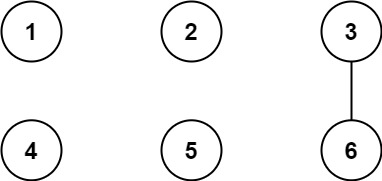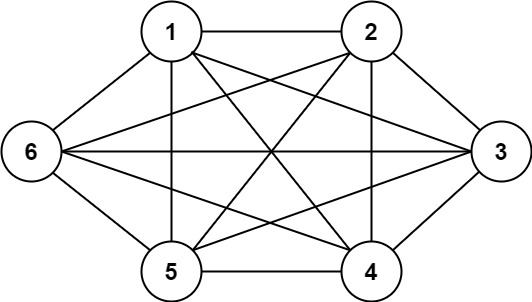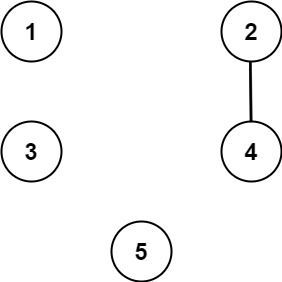##### Welcome to Subscribe On Youtube

Formatted question description: https://leetcode.ca/all/1627.html

# 1627. Graph Connectivity With Threshold (Hard)

We have n cities labeled from 1 to n. Two different cities with labels x and y are directly connected by a bidirectional road if and only if x and y share a common divisor strictly greater than some threshold. More formally, cities with labels x and y have a road between them if there exists an integer z such that all of the following are true:

• x % z == 0,
• y % z == 0, and
• z > threshold.

Given the two integers, n and threshold, and an array of queries, you must determine for each queries[i] = [ai, bi] if cities ai and bi are connected (i.e. there is some path between them).

Return an array answer, where answer.length == queries.length and answer[i] is true if for the ith query, there is a path between ai and bi, or answer[i] is false if there is no path.

Example 1:Input: n = 6, threshold = 2, queries = [[1,4],[2,5],[3,6]]
Output: [false,false,true]
Explanation: The divisors for each number:
1:   1
2:   1, 2
3:   1, 3
4:   1, 2, 4
5:   1, 5
6:   1, 2, 3, 6
Using the underlined divisors above the threshold, only cities 3 and 6 share a common divisor, so they are the
only ones directly connected. The result of each query:
[1,4]   1 is not connected to 4
[2,5]   2 is not connected to 5
[3,6]   3 is connected to 6 through path 3--6


Example 2:Input: n = 6, threshold = 0, queries = [[4,5],[3,4],[3,2],[2,6],[1,3]]
Output: [true,true,true,true,true]
Explanation: The divisors for each number are the same as the previous example. However, since the threshold is 0,
all divisors can be used. Since all numbers share 1 as a divisor, all cities are connected.


Example 3:Input: n = 5, threshold = 1, queries = [[4,5],[4,5],[3,2],[2,3],[3,4]]
Output: [false,false,false,false,false]
Explanation: Only cities 2 and 4 share a common divisor 2 which is strictly greater than the threshold 1, so they are the only ones directly connected.
Please notice that there can be multiple queries for the same pair of nodes [x, y], and that the query [x, y] is equivalent to the query [y, x].


Constraints:

• 2 <= n <= 104
• 0 <= threshold <= n
• 1 <= queries.length <= 105
• queries[i].length == 2
• 1 <= ai, bi <= cities
• ai != bi

Related Topics:
Math, Union Find

## Solution 1. Union Find

Intuition:

1. If two numbers share the same factor, use union find to connect them.
2. For each query, use union find to check if they are connected.

For #1, the brute force way is to check all combination pairs of cities which is O(N^2) time complexity and will get TLE.

A more efficient way is to use similar idea as in Sieve of Eratosthenes.

For each number i in [1 + threshold, n], we connect i with multiples of i (i.e. 2 * i, 3 * i, 4 * i, ...).

In the worst case where threshold = 0, iterating all the city pairs cost N + N / 2 + N / 3 + ... + 1 = N * (1 + 1 / 2 + 1 / 3 + ... + 1 / N). 1 + 1 / 2 + 1 / 3 + ... + 1 / N is a harmonic series and bounded by logN. So the iteration takes O(NlogN).

// OJ: https://leetcode.com/problems/graph-connectivity-with-threshold/
// Time: O(NlogN + Q)
// Space: O(N)
// Ref: https://leetcode.com/problems/graph-connectivity-with-threshold/discuss/899595/C%2B%2BJavaPython-Union-Find-O(N-*-logN-%2B-q)
class UnionFind {
vector<int> id;
public:
UnionFind(int n) : id(n) {
iota(begin(id), end(id), 0);
}
int find(int x) {
return id[x] == x ? x : (id[x] = find(id[x]));
}
void connect(int a, int b) {
int p = find(a), q = find(b);
if (p == q) return;
id[p] = q;
}
bool connected(int a, int b) {
return find(a) == find(b);
}
};
class Solution {
public:
vector<bool> areConnected(int n, int threshold, vector<vector<int>>& Q) {
unordered_map<int, int> m;
UnionFind uf(n);
for (int i = 1 + threshold; i <= n; ++i) {
for (int j = 2 * i; j <= n; j += i) {
uf.connect(i - 1, j - 1);
}
}
vector<bool> ans;
for (auto &q : Q) ans.push_back(uf.connected(q - 1, q - 1));
return ans;
}
};

• class Solution {
public List<Boolean> areConnected(int n, int threshold, int[][] queries) {
int[] parents = new int[n + 1];
for (int i = 1; i <= n; i++)
parents[i] = i;
int max = n / 2;
for (int i = threshold + 1; i <= max; i++) {
for (int j = i * 2; j <= n; j += i)
union(parents, i, j);
}
List<Boolean> list = new ArrayList<Boolean>();
for (int[] query : queries)
return list;
}

public void union(int[] parents, int index1, int index2) {
parents[find(parents, index1)] = find(parents, index2);
}

public int find(int[] parents, int index) {
while (parents[index] != index) {
parents[index] = find(parents, parents[index]);
index = parents[index];
}
return index;
}
}

• // OJ: https://leetcode.com/problems/graph-connectivity-with-threshold/
// Time: O(NlogN + Q)
// Space: O(N)
// Ref: https://leetcode.com/problems/graph-connectivity-with-threshold/discuss/899595
class UnionFind {
vector<int> id;
public:
UnionFind(int n) : id(n) {
iota(begin(id), end(id), 0);
}
int find(int x) {
return id[x] == x ? x : (id[x] = find(id[x]));
}
void connect(int a, int b) {
id[find(a)] = find(b);
}
bool connected(int a, int b) {
return find(a) == find(b);
}
};
class Solution {
public:
vector<bool> areConnected(int n, int threshold, vector<vector<int>>& Q) {
UnionFind uf(n + 1);
for (int d = threshold + 1; d <= n / 2; ++d) { // Enumerate all possible common divisors
for (int i = 2 * d; i <= n; i += d) { // Union all the numbers sharing the same divisors together
uf.connect(d, i);
}
}
vector<bool> ans;
for (auto &q : Q) ans.push_back(uf.connected(q, q));
return ans;
}
};